# Write an Enigma machine in Python

2022-02-02 06:26:08

Head author ：Bob Lord, link ：commons.wikimedia.org/w/index.php… CC BY-SA 3.0 Agreement authorization . therefore , This paper also adopts this protocol .

## cause

One day , In my spare time , I came across a video ：

【 Computer natural history 】 War code （ Part I ） How to recreate an engema machine _ Bili, Bili _bilibili

For enigma （Enigma） machine , Although I've heard of it , But there is no in-depth understanding of . So I ordered in .

The encryption method of this machine is too subtle , So I came up with the idea of reproducing it in programming language .

## Briefly, Enigma machine

Simply speaking , Enigma cipher machine is an encryption and decryption machine developed by Germany . During the second world war , It was used on a large scale by the German army .

The encryption method of this machine is very exquisite ： The encryption components are several rotors and a reflector . Put aside the knowledge of electricity ：

1. A letter goes into a rotor , Will come out of another letter , It's equivalent to changing a letter ;
2. Press a letter , The rightmost rotor will turn one grid , It will also drive other rotors , The principle is very similar to that of a clock ;
3. Because the rotor will rotate , Letters coming out of one rotor go into front of another rotor , The letters will also change ;
4. To the reflector , The letter will be replaced by another letter , Then reverse through the rotor , It is equivalent to encrypting again ;
5. The order and initial position of the rotor can be customized .

It can be seen that , Its encryption method is equivalent to setting a changed password table for each letter in the text , One letter for a password table . In theory, , A three rotor enigma cipher machine has 26 × 25 × 26 = 16900 A combination of （ Although it is actually slightly less than this number ）.

in addition , Some machines also have terminal blocks . Insert the two letter socket , It's equivalent to exchanging two letters . thus , There are more combinations .

It has the following properties ：

• Enter the same , rotor 、 The terminal block configuration is different , The output may be different ;
• Input is not equal to output ;
• rotor 、 When the terminal block configuration is the same , Enter ciphertext , Output the original text .

In short , You first write down the installation method and initial position of the rotor , Press the same button continuously , Write down the output . You'll find that , The output letters are different , But it can never be the letter entered . Turn the rotor back , Enter the last ciphertext , You'll find that , The output is all that letter .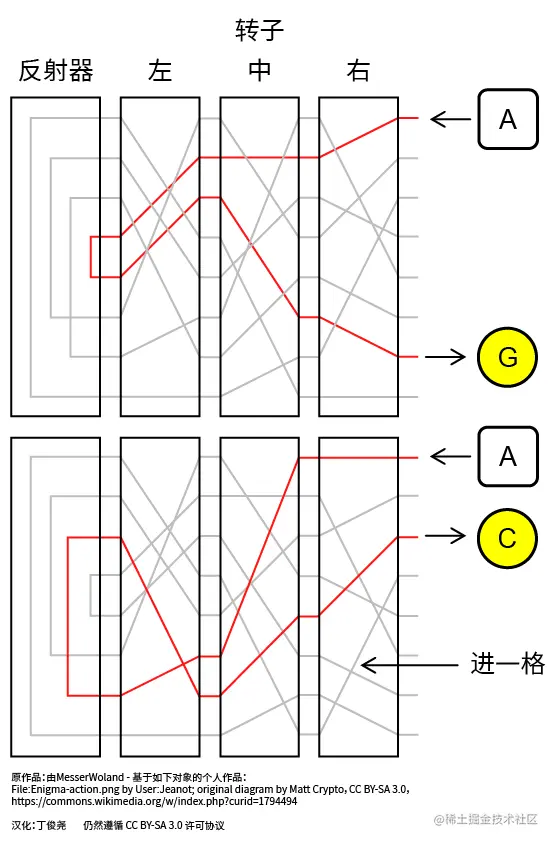Because of this nature , Intelligence agents in Britain and France think it can't be cracked , But for a defeated country in World War I , They don't care ; Polish intelligence once found the direction of decoding , However, due to the update of encryption methods and the flash war in Germany , Nor did it change the fate of Poland ; Last , A group of Britons including Turing , Just cracked it well , It also changed the direction of World War II .

## Define each class

An Enigma machine , There's input 、 Output 、 Fixed interface 、 rotor 、 It is composed of reflector and terminal block .

Input and output are very simple , Just use the incoming parameters and return values of the method .

Fixed interface 、 rotor 、 The structure of the reflector is very similar , Can be simulated as an input of a letter 、 A device that outputs a letter , At least the corresponding table and location information are required . For rotors , It turns one grid at a time , Change its position , The position can also be adjusted manually . therefore , We can create a class , Including these three , Name it `Plate`（ The disk ）. Then create an inheritance from `Plate` Three classes of .

Every time the terminal block is connected , Will connect several two letters in the alphabet .

As for the relationship between each component and Enigma machine , Because some models of Enigma machines don't have terminal blocks ; If there is a terminal block , Then it is an integral part of the machine , So the terminal block is initialized together when the Enigma machine is initialized . As for the disc , The fixed interface is initialized together when the Enigma machine is initialized , The rotor and reflector are initialized before .

thus , We can initially define the following structure ：

``````class Plate:
""" The disk , Including fixed interface 、 rotor 、 Reflector """
def __init__(self, map_table, position): ...
def encrypt(self, letter): ...  #  Encrypt a single letter

class EntryPlate(Plate):
""" Fixed interface """
...

class Rotor(Plate):
""" rotor """
def __init__(self, map_table, position): ...
def forward(self): ...  #  turn

class Reflector(Plate):
""" Reflector """
...

class Plugboard:
""" Wiring board """
def __init__(self, parent): ...
def plug(self, letter_1, letter_2): ...     #  connection
def unplug(self, letter): ...   #  Remove the wiring

class Enigma:
""" The Enigma machine """
def __init__(self, rotor_list, reflector): ...
#  The terminal block is initialized inside during the initialization of Enigma machine , Therefore, it is not introduced into
def input(self, string): ...    #  Input string
def set_position(self, *position_list): ...     #  Set the position of each rotor
Copy code ``````

Of course , Each class initialization requires more parameters than this . and , These classes are actually distributed in different files , I put them together just for the convenience of explanation .

## Realize the function of disc

The basic function of the disc , Is to enter a letter , Output another letter .

The general Enigma machine only processes capital letters . In order to realize the function , When initializing, pass in `map_table` As A-Z The result of the transformation .

To explore how to transform , We can make a simple machine first , Only ASDF Four letters ：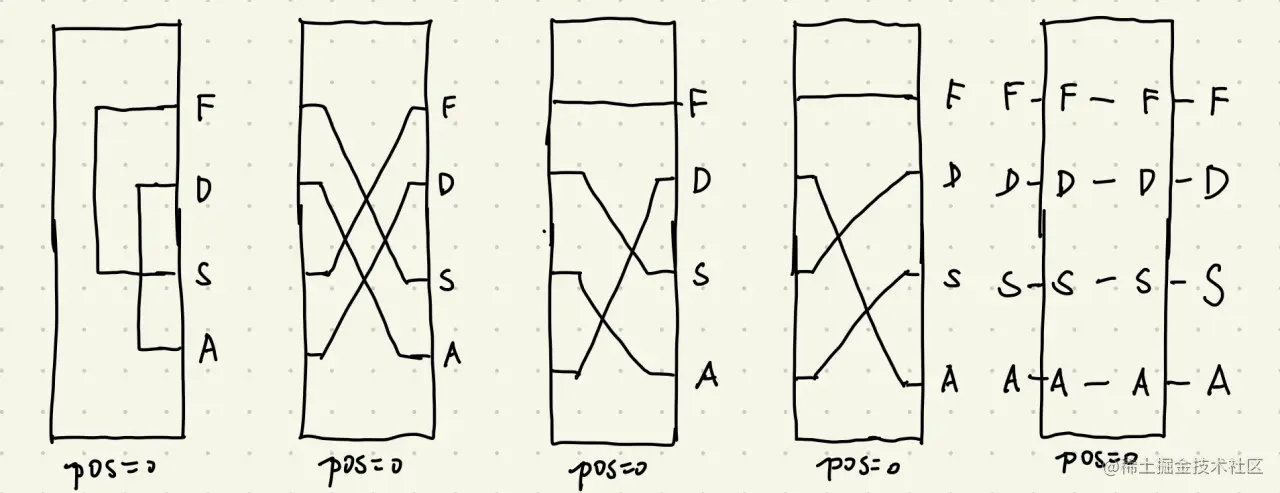In the initial position , Input A, The circuit is shown in the figure ：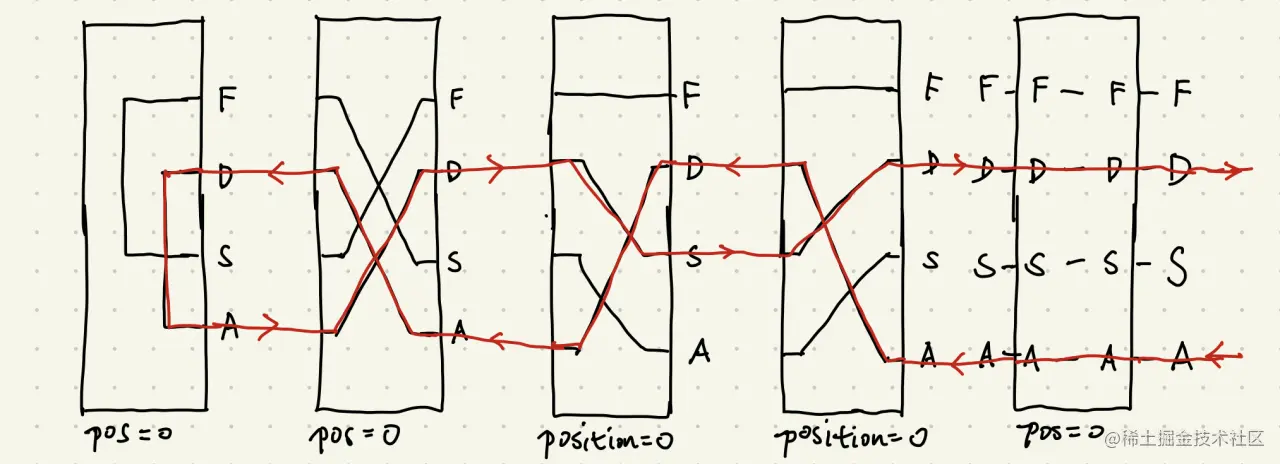Be careful ： We're just thinking about how to write the code , In fact, there are some noticeable differences between the principle of Enigma machine and the current simplified model , I'll explain later .

then , The rightmost rotor rotates up one grid , Input A, The circuit is shown in the figure ：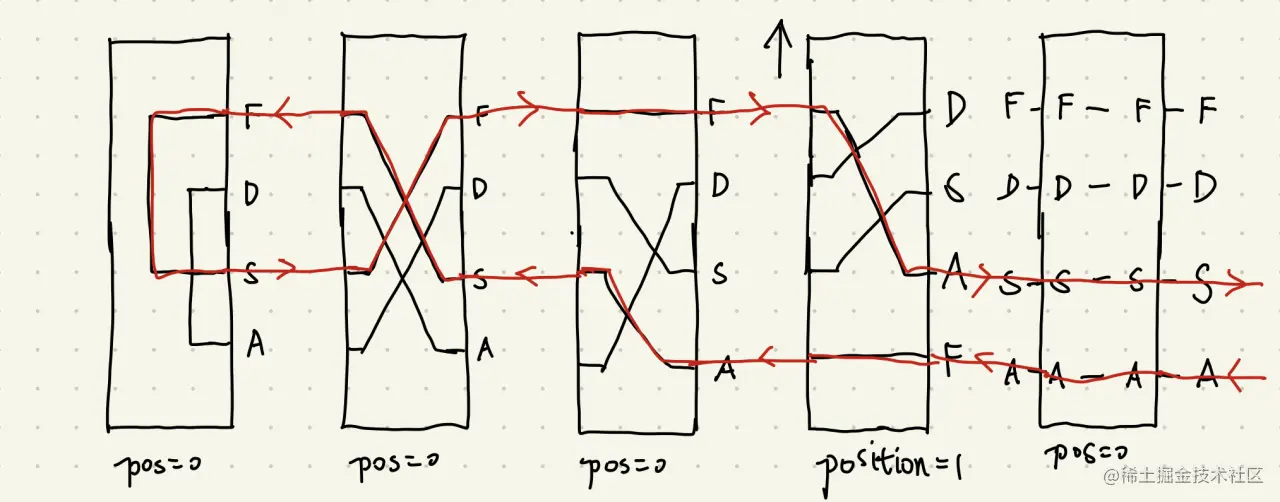First compare the two inputs , The current flows through the line of the rightmost rotor ：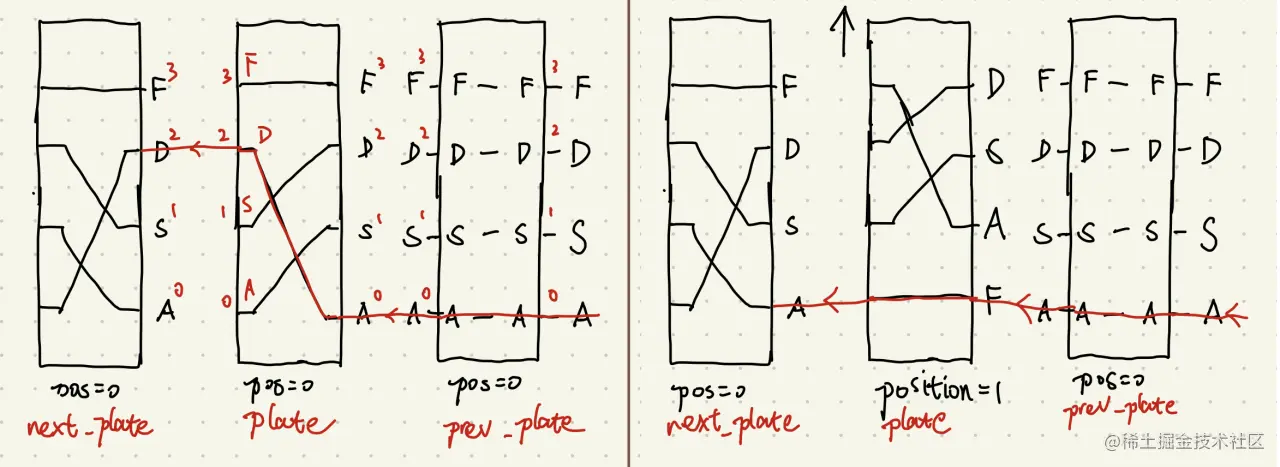It's not hard to see , You can first write a method to represent the current state of the rotor , Then use this method when encrypting .

When initializing the rotor , We will enter the input symbol of the rotor （`map_source`） And output results （`map_table`）, And its front and rear discs （`next_plate`, `prev_plate`）：

``````def __init__(self, map_source, map_table, prev_plate=None, next_plate=None):
self.map_source = map_source
dict_table = {}
for input_letter_index, input_letter in enumerate(map_source):
dict_table[input_letter] = map_table[input_letter_index]
self.dict_table = dict_table
self.prev_plate = prev_plate
self.next_plate = next_plate
self.position = 0
Copy code ``````

`self.dict_table` It expresses the corresponding relationship between input and output . For the rightmost rotor , as follows ：

``````{'A': 'D', 'S': 'A', 'D': 'S', 'F': 'F'}
Copy code ``````

Try to output `self.position` Before and after the change , The state of the rotor （`current_state`）：

``````@property
def current_state(self):
right = list(self.dict_table.keys())
left = list(self.dict_table.values())
current_right = right[-self.position:] + right[:-self.position]
current_left = left[-self.position:] + left[:-self.position]
return {'left': current_left, 'right': current_right}
# self.position == 0: {'left': ['S', 'D', 'A', 'F'], 'right': ['A', 'S', 'D', 'F']}
# self.position == 1: {'left': ['F', 'S', 'D', 'A'], 'right': ['F', 'A', 'S', 'D']}
Copy code ``````

We can notice that , The letter when the current enters the rotor , The output of the previous disc is not necessarily the same . So we also need to find the letter when entering the rotor ：

``````def transform_letter(self, letter):
letter_index = self.prev_plate.current_state['right'].index(letter)
transformed_letter = self.current_state['right'][letter_index]
Copy code ``````

Then encrypt ：

``````def encrypt(self, letter):
return self.dict_table[self.transform_letter(letter)]
Copy code ``````

The above method can always use electric current to get out of the reflector . however , If the current comes out of the reflector , It's about to change the code . This time we don't have to `next_plate` and `prev_plate`, Instead, use `left_plate` and `right_plate`, Lest you get confused next . By the way, consider the leftmost and rightmost situations .

in addition , By the way, add the method of adjusting the position ：

``````def __init__(self, map_source, map_table, left_plate=None, right_plate=None):
self.map_source = map_source
dict_table = {}
for input_letter_index, input_letter in enumerate(map_source):
dict_table[input_letter] = map_table[input_letter_index]
self.dict_table = dict_table
self.left_plate = left_plate
self.right_plate = right_plate
self.position = 0

def transform_letter(self, letter, from_plate):
letter_index = from_plate.current_state['right'].index(letter)
transformed_letter = self.current_state['right'][letter_index]

def encrypt(self, letter, letter_from='right'):
if letter_from == 'left':
from_plate = self.left_plate
else:
from_plate = self.right_plate
if from_plate:
letter = self.transform_letter(letter, from_plate)
if letter_from == 'left':
key_index = list(self.dict_table.values()).index(letter)
left_letter = list(self.dict_table.keys())[key_index]
return left_letter
else:
return self.dict_table[letter]

def set_position(self, position):
position_int = 0
if isinstance(position, str):
if position in self.map_source:
position_int = self.map_source.index(position)
elif isinstance(position, int):
if 0 <= position < len(self.map_source):
position_int = position
self.position = position_int
Copy code ``````

The above is placed in `Plate` Methods in class . Next , On demand configuration `auto_rotatable` Properties of ：

``````class Plate:
def __init__(self, map_source, map_table, left_plate=None, right_plate=None, auto_rotatable=False):
self.map_source = map_source
dict_table = {}
for input_letter_index, input_letter in enumerate(map_source):
dict_table[input_letter] = map_table[input_letter_index]
self.dict_table = dict_table
self.left_plate = left_plate
self.right_plate = right_plate
self.position = 0
self.auto_rotatable = auto_rotatable

class Rotor(Plate):
def __init__(self, map_source, map_table, left_plate=None, right_plate=None, ...):
super().__init__(map_source, map_table, left_plate, right_plate, auto_rotatable=True)

class Reflector(Plate):
def __init__(self, map_source, map_table, right_plate=None, ...):
super().__init__(map_source, map_table, left_plate=None, right_plate=right_plate, auto_rotatable=False)

class EntryPlate(Plate):
def __init__(self, map_source, map_table, left_plate=None, ...):
super().__init__(map_source, map_table, left_plate, right_plate=None, auto_rotatable=False)
Copy code ``````

Set this property , It's for you `Rotor` Class add method `forward()`, Turn one grid , Along with processing carry ：

``````def forward(self):
original_position = self.position
if original_position == len(self.map_source) - 1:
self.position = 0
else:
self.position += 1
if self.left_plate and self.left_plate.position == len(self.map_source) - 1:
if self.left_plate.auto_rotatable:
self.left_plate.forward()
return self.position
Copy code ``````

The part of the disc is over . Let's start building the terminal block .

## Build the terminal block

The construction of the terminal block is relatively simple , Is to create a correspondence table .

``````class Plugboard:
""" Wiring board """
def __init__(self):
self.map_dict = {}

def plug(self, letter_1, letter_2):
self.map_dict[letter_1] = letter_2
self.map_dict[letter_2] = letter_1

def unplug(self, letter):
another_letter = self.map_dict[letter]
del self.map_dict[letter]
del self.map_dict[another_letter]
Copy code ``````

## Assemble Enigma machine

Due to the initialization sequence mentioned earlier , When we initialize the Enigma machine , Do the following ：

1. List of incoming rotors （ The rotors in this list are from right to left ）、 Reflector ;
2. Define the disk on the right side of the reflector as the leftmost rotor ;
3. Define fixed interface ;
4. Define the left and right discs of each rotor ;
5. Initialize the patch panel .

So there is the following code ：

``````class Enigma:
def __init__(self, rotor_list, reflector):
self.rotors = rotor_list
self.reflector = reflector
self.reflector.right_plate = self.rotors[-1]
self.entry_plate = EntryPlate(
map_source=self.reflector.map_source, map_table=self.reflector.map_source, left_plate=self.rotors
)
for rotor_index, rotor in enumerate(self.rotors):
if rotor_index < len(self.rotors) - 1:
rotor.left_plate = self.rotors[rotor_index + 1]
else:
rotor.left_plate = self.reflector
if rotor_index > 0:
rotor.right_plate = self.rotors[rotor_index - 1]
else:
rotor.right_plate = self.entry_plate
self.plugboard = Plugboard()
Copy code ``````

Let's write down the method of setting the position of each rotor `set_position()`. If no parameter is filled in , Set to 0（ We define the initial position as 0, This is different from the actual Enigma machine , In this case, the corresponding number on the rotor is 01）：

``````def set_position(self, *position_list):
if not position_list:
position_list = (0, ) * len(self.rotors)
elif len(position_list) != len(self.rotors):
raise AttributeError('Position list length is not equal to rotors number')
for rotor, position in zip(self.rotors, position_list):
rotor.set_position(position)
Copy code ``````

Then there is the input method `input()`. Finally, it's the last link , But there's not much to say , The previous principle is quite clear .

``````def input(self, string):
new_string_list = []
for letter in string:
if letter in self.plugboard.map_dict:
letter = self.plugboard.map_dict[letter]
for rotor in self.rotors:
letter = rotor.encrypt(letter, 'right')
letter = self.reflector.encrypt(letter, 'right')
for rotor in reversed(self.rotors):
letter = rotor.encrypt(letter, 'left')
letter = self.entry_plate.encrypt(letter, 'left')
if letter in self.plugboard.map_dict:
letter = self.plugboard.map_dict[letter]
new_string_list.append(letter)
self.rotors.forward()
return ''.join(new_string_list)
Copy code ``````

## test

Then let's try to run ！ Don't tube the patch panel yet .

``````if __name__ == '__main__':
main_map_source = 'ASDF'
e = Enigma(
[
Rotor(map_source=main_map_source, map_table='DASF'),
Rotor(map_source=main_map_source, map_table='SDAF'),
Rotor(map_source=main_map_source, map_table='DFAS'),
],
Reflector(map_source=main_map_source, map_table='DFAS')
)
print(e.input('AA'))     # 'DS'
Copy code ``````

Output ：

``````DS
Copy code ``````

As expected .

however , The previous simplified model is a simplified model after all , Let's find a practical example .

I found a simulator of Enigma machine ：Enigma Simulator. It comes with file , This will better restore the real situation .

However, the connection method mentioned in the document is not convenient for input ：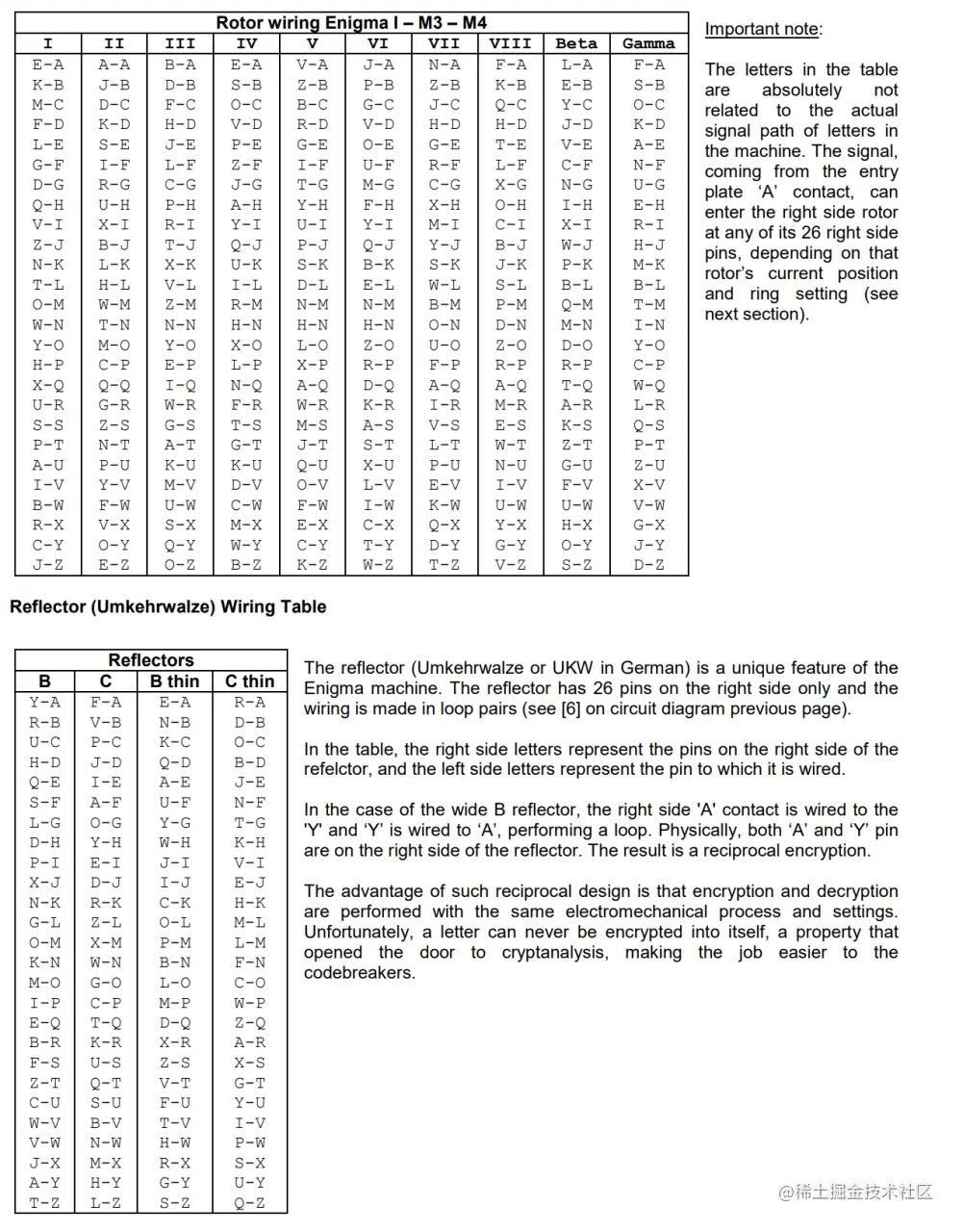But I found another page about Enigma machine ：Enigma Rotor and Umkehrwalze Wirings, It puts the connection mode in one line , Easier to enter .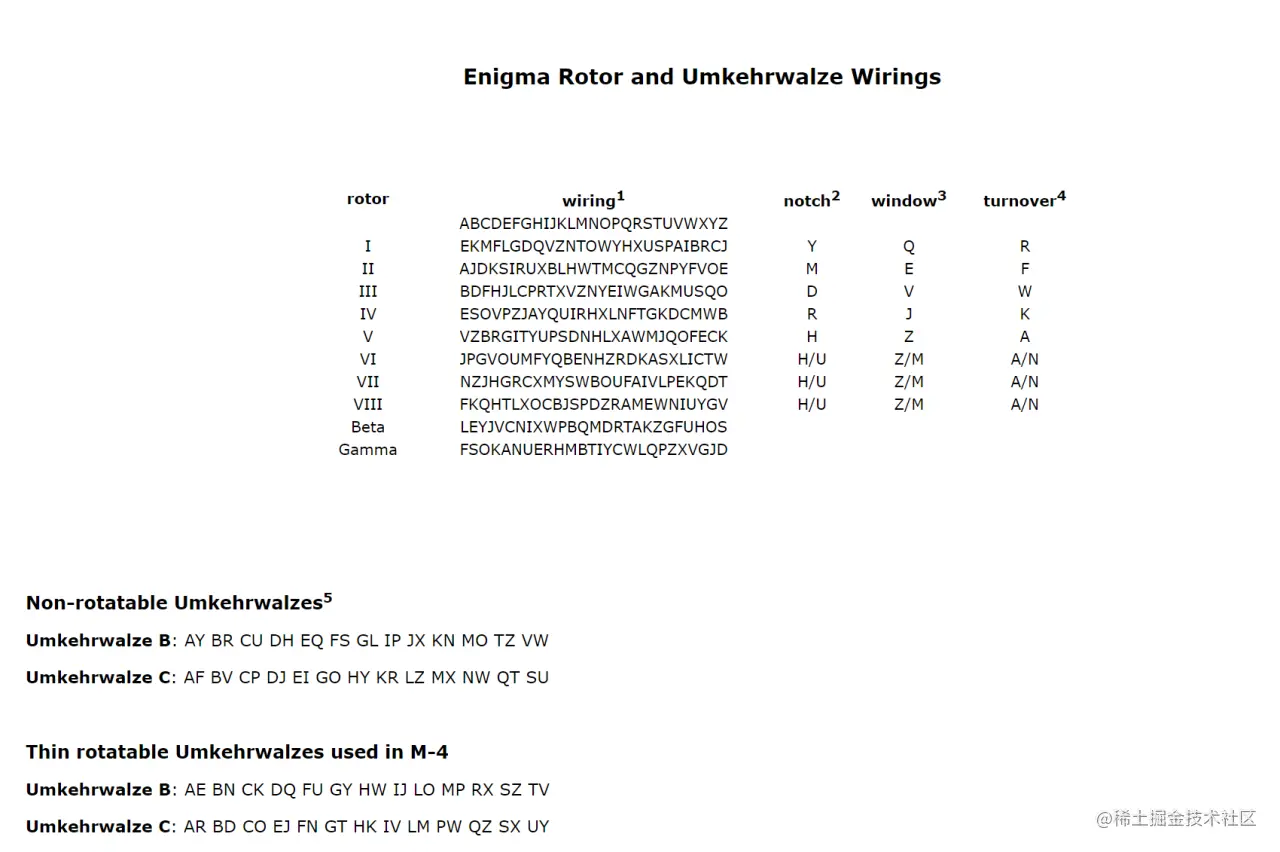then ：

``````if __name__ == '__main__':
main_map_source = 'ABCDEFGHIJKLMNOPQRSTUVWXYZ'
e = Enigma(
[
Rotor(map_source=main_map_source, map_table='BDFHJLCPRTXVZNYEIWGAKMUSQO'),
Rotor(map_source=main_map_source, map_table='AJDKSIRUXBLHWTMCQGZNPYFVOE'),
Rotor(map_source=main_map_source, map_table='EKMFLGDQVZNTOWYHXUSPAIBRCJ'),
],
Reflector(map_source=main_map_source, map_table='YRUHQSLDPXNGOKMIEBFZCWVJAT')
)
print(e.input('AAAAAAAAAAAAAAAAAAAAAAAAAAAAAA'))
Copy code ``````

Output results ：

``````UOBEFMBPLDCMTBSKTLXCWOGZDBUOBE
Copy code ``````

Compare the results with the simulator ：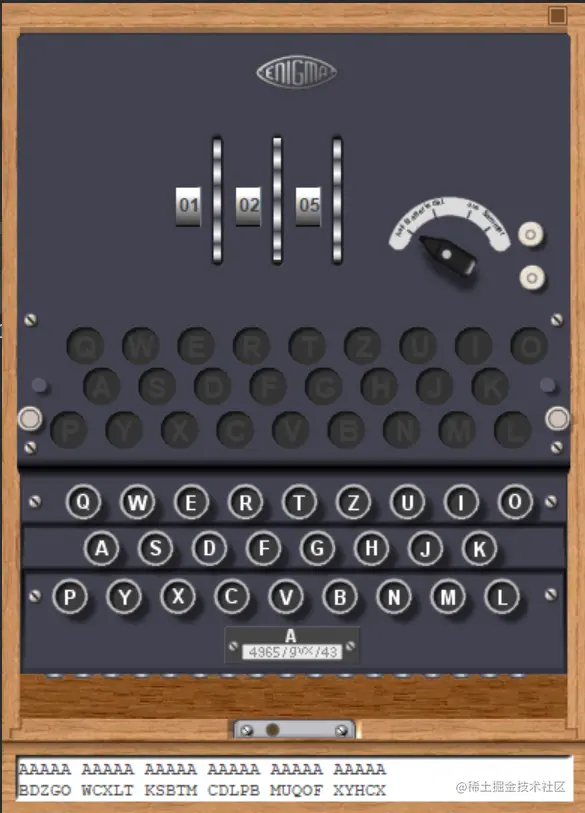``````BDZGOWCXLTKSBTMCDLPBMUQOFXYHCX
Copy code ``````

It can't be as like as two peas , At least it's irrelevant .

What's going on ？

## Several points that cannot be ignored

In fact, when writing programs , I don't know how many pits I stepped on . The first is how to judge the direction from the output of the previous disc to the input of the next disc . It only says the results , But it took me three days , Only from the A website Inspired by ：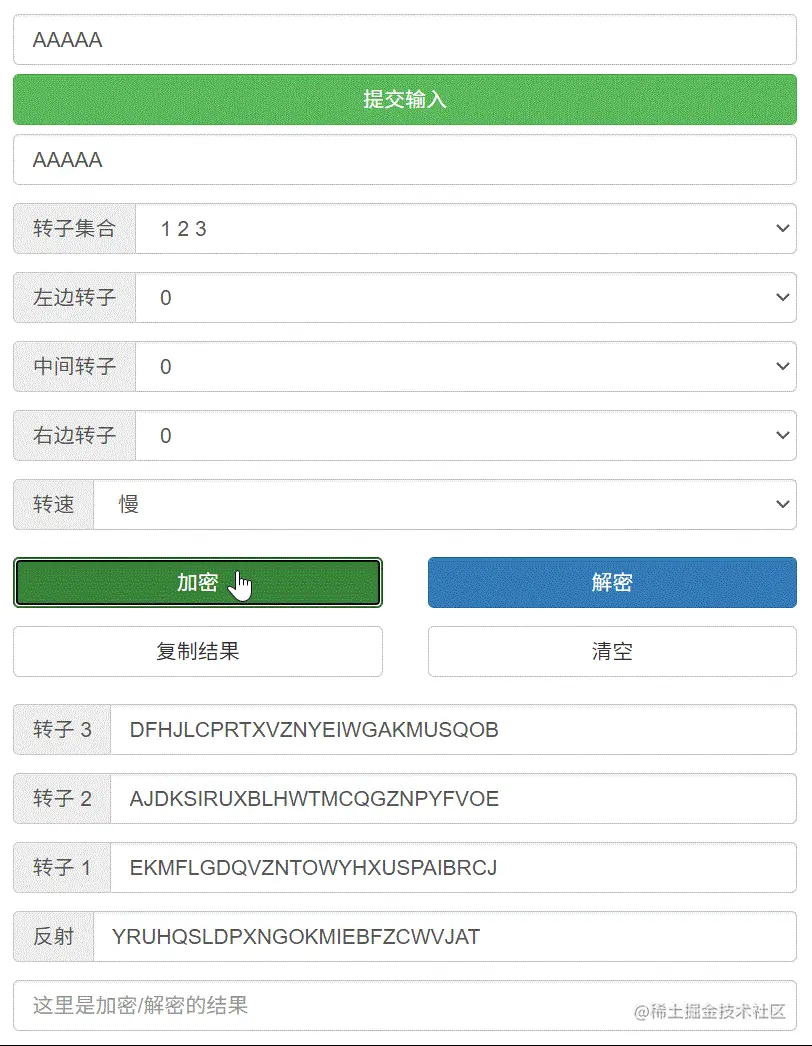Then the output mentioned before is inconsistent .

Browsing Enigma code machine - Wikipedia , An encyclopedia of freedom when , I found a sentence in it ：

When you press a key , The rotor will turn before the circuit is connected .

in other words , First turn the rotor , Then turn on the circuit .

So change the code , Adjust the rotor rotation command before translation , Run again , Output ：

``````OBEFMBPLDCMTBSKTLXCWOGZDBUOBEF
Copy code ``````

It's still different . Don't worry. , We open the cover of the simulator ：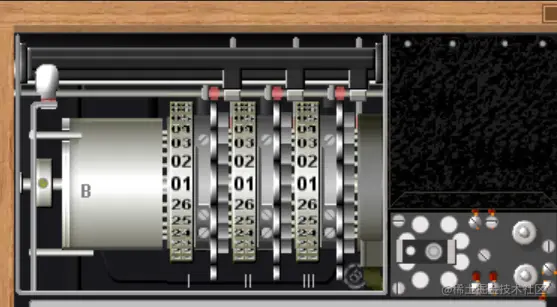You can find , From this perspective , The rotor of Enigma machine rotates downward . And the picture we drew before , It turns upward .

This is more troublesome . I want to be compatible with the previous situation , So modify the code ：

``````class Plate:
def __init__(self, map_source, map_table, left_plate=None, right_plate=None, auto_rotatable=False, rotate_up=False):
...
self.rotate_up = rotate_up
...
@property
def current_state(self):
right = list(self.dict_table.keys())
left = list(self.dict_table.values())
transform_position = (-2 * self.rotate_up + 1) * self.position
current_right = right[transform_position:] + right[:transform_position]
current_left = left[transform_position:] + left[:transform_position]
return {'left': current_left, 'right': current_right}
Copy code ``````

Inside `-2 * self.rotate_up + 1`, When `self.rotate_up` by `True` when , The value is `-1`; When `self.rotate_up` by `False` when , The value is `1`.

Run again ：

``````BDZGOWCXLTKSBTMCDLPBMFEBOUBDZG
Copy code ``````

It looks right . wait ：

``````BDZGOWCXLTKSBTMCDLPBMFEBOUBDZG  #  Code
BDZGOWCXLTKSBTMCDLPBMUQOFXYHCX  #  Simulator
Copy code ``````

From 22 A letter starts , Not so . What's going on ？

Take a look at the simulator ：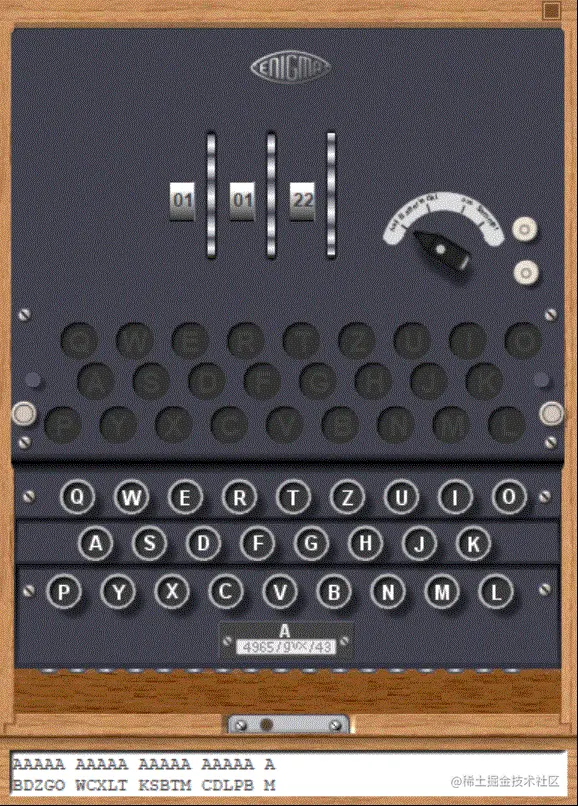You see ？ Carry is not carried at the end of a turn . As for how to carry , The documents mentioned before are ：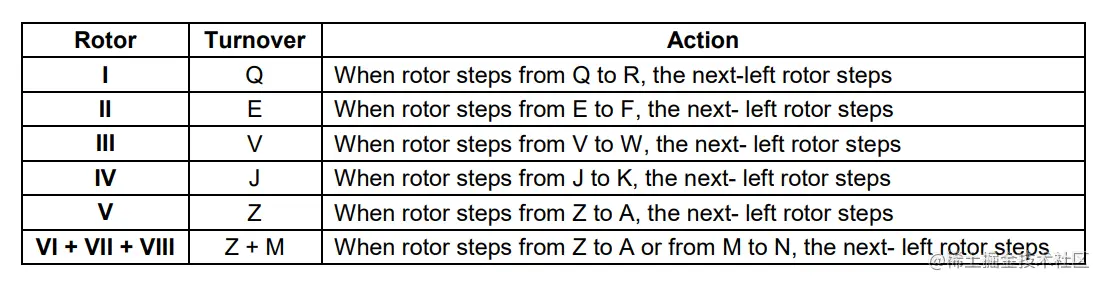Change it .

``````class Plate:
def __init__(self, map_source, map_table, left_plate=None, right_plate=None, auto_rotatable=False, rotate_up=False):
self.map_source = map_source
dict_table = {}
for input_letter_index, input_letter in enumerate(map_source):
dict_table[input_letter] = map_table[input_letter_index]
self.dict_table = dict_table
self.left_plate = left_plate
self.right_plate = right_plate
self.position = 0
self.auto_rotatable = auto_rotatable
self.rotate_up = rotate_up
self.turnover = []

class Rotor(Plate):

def turnover_single_check_and_standardize(self, turnover_single, map_source):
if isinstance(turnover_single, str):
return map_source.index(turnover_single)
elif isinstance(turnover_single, int):
return turnover_single

def __init__(self, map_source, map_table, left_plate=None, right_plate=None, rotate_up=False, turnover=None):
super().__init__(map_source, map_table, left_plate, right_plate, auto_rotatable=True, rotate_up=rotate_up)
if turnover is None:
turnover = map_source[-1]
if isinstance(turnover, list) or isinstance(turnover, tuple):
turnover_list = []
for turnover_item in turnover:
turnover_list.append(self.turnover_single_check_and_standardize(turnover_item, map_source))
else:
turnover_list = [self.turnover_single_check_and_standardize(turnover, map_source)]
self.turnover = turnover_list

def forward(self):
original_position = self.position
if original_position == len(self.map_source) - 1:
self.position = 0
else:
self.position += 1
if original_position in self.turnover:
if self.left_plate:
if self.left_plate.auto_rotatable:
self.left_plate.forward()
return self.position
Copy code ``````

function , Compared with the results of the simulator ：

``````BDZGOWCXLTKSBTMCDLPBMFEBOXYHCX  #  Code
BDZGOWCXLTKSBTMCDLPBMUQOFXYHCX  #  Simulator
Copy code ``````

There is a small difference in the middle . Oh , The rotor tested is not set to carry , Re reform ：

``````if __name__ == '__main__':
main_map_source = 'ABCDEFGHIJKLMNOPQRSTUVWXYZ'
e = Enigma(
[
Rotor(map_source=main_map_source, map_table='BDFHJLCPRTXVZNYEIWGAKMUSQO', turnover='V'),
Rotor(map_source=main_map_source, map_table='AJDKSIRUXBLHWTMCQGZNPYFVOE', turnover='E'),
Rotor(map_source=main_map_source, map_table='EKMFLGDQVZNTOWYHXUSPAIBRCJ', turnover='Q'),
],
Reflector(map_source=main_map_source, map_table='YRUHQSLDPXNGOKMIEBFZCWVJAT')
)
print(e.input('AAAAAAAAAAAAAAAAAAAAAAAAAAAAAA'))
Copy code ``````

function ：

``````BDZGOWCXLTKSBTMCDLPBMUQOFXYHCX  #  Code
BDZGOWCXLTKSBTMCDLPBMUQOFXYHCX  #  Simulator
Copy code ``````

It's finally the same .

Try to see if the output results can return to the original results ：

``````if __name__ == '__main__':
main_map_source = 'ABCDEFGHIJKLMNOPQRSTUVWXYZ'
e = Enigma(
[
Rotor(map_source=main_map_source, map_table='BDFHJLCPRTXVZNYEIWGAKMUSQO', turnover='V'),
Rotor(map_source=main_map_source, map_table='AJDKSIRUXBLHWTMCQGZNPYFVOE', turnover='E'),
Rotor(map_source=main_map_source, map_table='EKMFLGDQVZNTOWYHXUSPAIBRCJ', turnover='Q'),
],
Reflector(map_source=main_map_source, map_table='YRUHQSLDPXNGOKMIEBFZCWVJAT')
)
print(e.input('AAAAAAAAAAAAAAAAAAAAAAAAAAAAAA'))
e.set_position()
print(e.input('BDZGOWCXLTKSBTMCDLPBMUQOFXYHCX'))
Copy code ``````

function ：

``````BDZGOWCXLTKSBTMCDLPBMUQOFXYHCX
AAAAAAAAAAAAAAAAAAAAAAAAAAAAAA
Copy code ``````

Everything is all right .

You can also test the results of inserting the patch panel . Because there's too much in the front , Let's leave it to you .

## project

actually , We also need to consider information such as the correctness of the input data . But it's not written here , Too much space . If you are interested, you can see me in GitHub Project on ：DingJunyao/myenigma: Python-based Enigma., Welcome to Fork、Star.

I've written the project into a Python package , You can install... From the following command line ：

``````pip install myenigma
Copy code ``````

Only support Python 3.10 Later versions , file ：myenigma - Acridine

Actually, I wanted to register as `enigma` Of , In limine stay PyPI No duplicate names were found on the . Until I came across A namesake , But I don't know why , It's not in the search record .